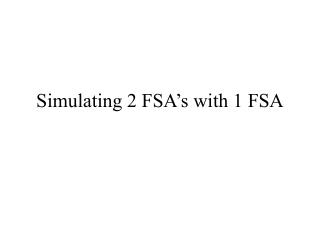Download PresentationSimulating 2 FSA’s with 1 FSA

# Simulating 2 FSA’s with 1 FSA - PowerPoint PPT PresentationDownload Presentation## Simulating 2 FSA’s with 1 FSA

- - - - - - - - - - - - - - - - - - - - - - - - - - - E N D - - - - - - - - - - - - - - - - - - - - - - - - - - -
##### Presentation Transcript

1. Simulating 2 FSA’s with 1 FSA

2. Purpose • This presentation presents an example execution of the algorithm which takes as input two FSA’s and produces as output an FSA which “simulates” both input FSA’s on any input string • Algorithm Specification • Input: FSA’s M1 and M2 • Output: FSA M3

3. a,b b a b a The two input FSA’s a,b a a b B I II a A D b b III C M1 M2

4. a,b b a b a Initialization a,b a a b B I II a A D b b III C The initial state of the output FSA M3 is the ordered pair of the initial states of M1 and M2.

5. a,b b a b a Expand State I,A a,b a a b B I II a A D b b III C

6. a,b b a b a Add New States a,b a a b B I II a A D b b III C

7. a,b b a b a Expand State I,C a,b a a b B I II a A D b b III C

8. a,b b a b a Add New States a,b a a b B I II a A D b b III C

9. a,b b a b a Expand State II,B a,b a a b B I II a A D b b III C

10. a,b b a b a Add One New State a,b a a b B I II a A D b b III C

11. a,b b a b a Expand State I,B a,b a a b B I II a A D b b III C

12. a,b b a b a No New States Added a,b a a b B I II a A D b b III C

13. a,b b a b a Expand State II,D a,b a a b B I II a A D b b III C

14. a,b b a b a Add One New State a,b a a b B I II a A D b b III C

15. a,b b a b a Expand State III,B a,b a a b B I II a A D b b III C

16. a,b b a b a No New States Added a,b a a b B I II a A D b b III C

17. a,b b a b a Expand State III,D a,b a a b B I II a A D b b III C

18. a,b b a b a Add 1 New State a,b a a b B I II a A D b b III C

19. a,b b a b a Expand State I,D a,b a a b B I II a A D b b III C

20. a,b b a b a No More New States a,b a a b B I II a A D b b III C

21. a,b a a a,b b b B I II a a b a A D b b III C Determining Final States Set Intersection Both input machines should accept in order for new machine to accept The one accepting state of the new machine M3 is I,D Set Union If either input machine accepts, new machine should accept The six accepting states of the new machine M3 are I,A; I,C; I,B; II,D; III,D; I,D Set Difference: M1 should accept but M2 should not The three accepting states of the new machine M3 are I,A; I,C; I,B Symmetric Difference: M1 should accept but M2 should not or M2 should accept but M1 should not The five accepting states of the new machine M3 are I,A; I,C; I,B; II,D; III,D